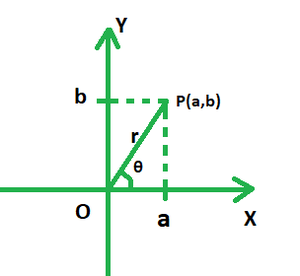# Find the modulus and the argument of 15 – 4i and a – ai where a > 0

• Last Updated : 29 Dec, 2021

Complex numbers are the summation of both real and imaginary parts, represented usually as a + ib, where i is called the imaginary part and is the square root of -1. Let’s learn about the modulus and argument of a complex number in detail in order to solve problems related to them,

### Modulus and Argument of a Complex Number

When a complex number is presented on a graph, its real part is plotted on the x-axis and the imaginary part on the y- axis. Say if the number were to be represented by point P in the figure given below, triangles OPA and OPB would be both right-angled. Clearly, in the right triangle POA, PO is the hypotenuse; Oa is the base and Pa is the perpendicular. Using Pythagoras Theorem, we have:

OP2 = OA2 + PA2

OP =The absolute value of a complex number is regarded as its modulus. It is the square root of the sum of squares of its real and imaginary parts. In the above case, OP is the modulus of the complex number of the form z = a + ib, and is denoted by r.

The argument of a complex number is defined as the angle at which the graph of the number is inclined towards the real axis. The argument of a complex number of the form z = a + ib is given as:

θ =### Find the modulus and the argument of 15 – 4i and a – ai where a > 0.

Solution:

Given: z1 = 15 – 4i

• Modulus =• Argument =Also, z2 = a – ai

• Modulus == √2a

• Argument == π/4.

### Similar Problems

Question 1: Find the modulus and argument of 1 + i.

Solution:

a = 1, b = 1

Modulus == √2

Argument = tan-1[1/1]

= tan-1[π/4]

= π/4

Question 2: Find the modulus and argument of -3 – 3i.

Solution:

a = -3, b = -3

Modulus == 3√2

Argument = tan-1[b/a]

= tan-1[3/3]

= π/4

Question 3: Find the modulus and argument of 2i.

Solution:

a = 0, b = 2

Modulus == √4

= 2

Argument = tan-1[2/0]

= π/2

Question 4: Find the modulus and argument of -4.

Solution:

a = -4, b = 0

Modulus == √16

= 4

Argument = tan-1[0/4]

= π

Question 5: Find the modulus and argument of -1 + 2i.

Solution:

a = -1, b = 2

Modulus == √5

Argument = tan-1[2/1]My Personal Notes arrow_drop_up